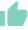# What is XIRR in Mutual Funds - Meaning, Use, Calculation, Difference with CAGRGaurav Seth
20 Likes | 772 days agoLikeShareGaurav Seth
Gurugram## XIRR MEANING

XIRR or Extended Internal Rate of Return is a dynamic and significantly used function in Excel/spreadsheet. It is used to calculate the personal returns of investors. In today’s time, where the markets are volatile, most investors opt to invest through SIP. So, multiple investments over different time periods can make the calculation of returns very complex and difficult since the more the variations, the more the purchase price varies. XIRR comes into the play here. XIRR gives you the present value of your investment and is a precisely calculated parameter which when applied to investments gives out a specific evaluation. XIRR can also be used in lump sum investments. In this article, we are going to explore more aspects of XIRR.

## XIRR IN MUTUAL FUNDS

As discussed, XIRR is a phenomenon used to calculate returns on investments where there are multiple installments at different time intervals. When you invest in different mutual funds, you are investing through lump sum or SIP, redeeming either through lump sum or SWP, XIRR is the feature that will help you to ascertain the returns by taking into consideration withdrawals and investments. Thus, it can be quoted that XIRR is an aggregation of multiple CAGRs.

## USE OF XIRR

Investment through SIP is considered ideal in many cases, but it is often seen that investments in the long term are irregular and the cash flows of the plan also vary. Sometimes, there are late deposits or early withdrawals, or sometimes, months in a row are skipped. In these cases, investors can’t rely on simple IRR.
Attention needs to be paid to factors like the amount of investment, the number of installments, and time intervals. These factors may determine the output differently with each investment. And this is the primary feature and function of XIRR, to provide you returns calculation by taking all the variations into consideration and presenting a consolidated return of a particular fund or scheme.

## CALCULATION OF XIRR

There are two broad ways to calculate XIRR.

First is by using EXCEL

The formula used here is =XIRR(values,dates,[guess])

Here,

Value is the array of values represented by reference to a range of cells that represent the series of cash flows.

Dates are a series of dates that correspond to the given fund. Subsequent dates should be later than the initial date.

[guess] is an initial guess or estimate of what the IRR can be. The default value taken by excel is 10%.

The process to use this is as follows:

1. Make two columns and title the left one with ‘DATE’ and list all the inflow/outflow dates.
2. Title the right column with “VALUES”. Write down the redeemed amounts in positive and the investment made in negative since they are outflows from you.
3. The last row of your sheet should contain the current value of your holding and the current date.
4. Just below it, use the XIRR function.
5. Now, you have to insert the respective value by referencing or typing corresponding to the respective date.
6. Now simply press enter and enjoy the precise analysis of your investments.

The second way

It is not much different but it rules out the guess part from the above process

The steps here are:

1. Transaction dates are listed on the left column.
2. Transaction values are written on the right column. Write down the redeemed amounts in positive and the investment made in negative since they are outflows from you.
3. The formula to be used here is “=XIRR(values,dates)*100

## DIFFERENCE BETWEEN CAGR AND XIRR

1. CAGR only considers the initial value, number of years, and the end value and does not consider multiple cash flows. Whereas, XIRR considers the multiple cash flows whether withdrawn or invested.
2. CAGR gives investors the compounded growth rate whereas XIRR is the average rate earned by each and every cash inflow/outflow made during the period.
3. XIRR is the measure of performance of cash flows whereas CAGR is the measure of performance of lump-sum investments.
4. XIRR gives the annualized returns whereas CAGR gives us the absolute return as it is an end-to-end return indicator.
5. Both the indicators will show different values if investors invest multiple times and also make withdrawals in the middle.
6. Both the indicators will show different values if the annual returns are not the same.

## SIMILARITIES BETWEEN CAGR AND XIRR

1. Both the indicators will show the same values if you invest lump sum only once and calculate them after one year.
2. Both the indicators will show the same values if investors are making multiple investments but the annual returns are the same throughout the tenure.

1. What is XIRR?

XIRR or Extended Internal Rate of Return is a dynamic and significantly used function in Excel/spreadsheet used to calculate the personal returns of investors when investing through SIP.

2. What is the use of XIRR?

The primary feature and function of XIRR are to provide you returns calculation by taking all the variations into consideration and presenting a consolidated return of a particular fund or scheme. It pays attention to factors like the amount of investment, the number of installments, and time intervals. These factors may determine the output differently with each investment.

3. What is the formula used in excel to calculate XIRR?

The formula of XIRR in excel is “=XIRR(values,dates,[guess])”

4. What is the key difference between CAGR and XIRR?

CAGR only considers the initial value, number of years, and the end value and does not consider multiple cash flows. Whereas, XIRR considers the multiple cash flows whether withdrawn or invested.